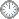# 也许该换个学法了...

This is an in-mail from TYUST.
新入の者--> What is going on ? (redirected)new

(接前：22 21 20) 命题 3.1 的证明.
Step 7. 第一段.
Let M = ψ*φ*uT.
---- uT 即 L.(参Step1)
.
Since Ω ≡ uT, the degree degHM = degH*φ*Ω) is bounded from above, by Step 3...
---- Ω ≡ uT ? ( 又见 “Since体” ).
---- degH*φ*Ω) = Hᵈ⁻¹*φ*Ω).
---- Step3: ψ*φ*Ω (系数)有上界.
(divisor 有上界盖指系数有上界 ?)
.
...which implies the coefficients of M are bounded from above.
---- 这句话显得多余.(?)
---- 因为 M 就是 ψ*φ*Ω.
.
In particular, we may assume T is exceptional over V, otherwise u would be bounded.
---- 这里实际上是按 T 是否 exceptional 做 “二分讨论”.
---- 若 T 非 exceptional, 则 u 有界 (待考).
(注: 本命题的目标是 u 有上界)
.
Thus M is exceptional over X, hence its support is inside Λ.
---- Supp M ⊂ Λ. (?)
T    M
V    X
.
So perhaps after replacing H we can assume H - M is ample.
---- perhaps 是何意 ?
.

---- 到此 “凑” 够了 Th1.6 的条件.
H      M
.
V      Δ
.

On the other hand, since T is ample, φ*uT ≤ ψ*M, by the negativity lemma, hence the coefficient of the birational transform of T in ψ*M is at least u.
---- M = ψ*φ*uT, 两边取ψ*, 得 ψ*M ≥ φ*uT.
(T ample 起到什么作用?)
---- 整理负引理出现的情境.
(更一般地可就若干主题做个整理).
.
Therefore, (V, Δ + 1/u M) is not klt as a(T, V, Δ + 1/u M) ≤ a(T, V, Δ) - 1 ≤ 0.
---- (V, Δ + 1/u M) klt 意味 a(T, V, Δ + 1/u M) > 0.
---- 怎么推导 a(T, V, Δ + 1/u M) ≤ a(T, V, Δ) - 1.
(T 的任意性来自何处?).
.
Now by Theorem 1.6, there is a positive number t depending only on d, eps', r such that (V, Δ + tM) is klt.
---- 第一段之后就可以用Th1.6, 但延迟到这里表述.
.
Therefore, t < 1/u, hence u < v: = 1/t.
---- 这里需要做点推导...
.

符号大全上下标.|| 常用：↑↓ π ΓΔΛΘΩμφΣ∈  ∪ ∩ ⊆ ⊇ ⊂ ⊃ ≤ ≥ ⌊ ⌋ ⌈ ⌉ ≠ ≡ ⁻⁰ ¹ ² ³ ᵈ ₀ ₁ ₂ ₃ ᵢ .

#### Glossary(AG)

*

Introduction
....
....
11/9
...
Proposition 5.5 11/5

http://blog.sciencenet.cn/blog-315774-1186477.html

## 全部精选博文导读

GMT+8, 2019-8-25 14:54

Powered by ScienceNet.cn

Copyright © 2007- 中国科学报社